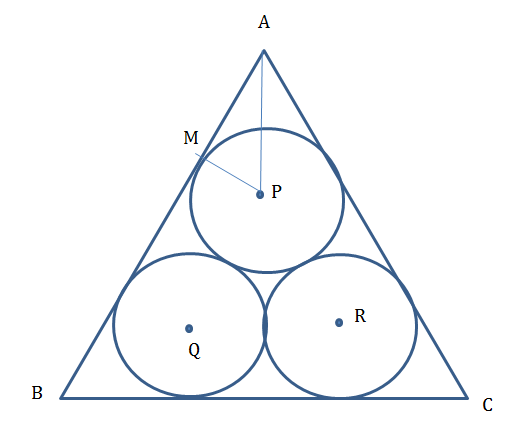# CAT Quantitative Aptitude Questions | Geometry Questions for CAT - Triangles

###### CAT Questions | CAT Geometry Questions | Triangles - Area

The question is from CAT Geometry - Triangles. In this problem, it discusses about ratio of areas three equal circles that is placed inside an equilateral triangle. CAT Geometry questions are heavily tested in CAT exam. Make sure you master Geometry problems.

Question 24: Three equal circles are placed inside an equilateral triangle such that any circle is tangential to two sides of the equilateral triangle and to two other circles. What is the ratio of the areas of one circle to that of the triangle

1. π : (6+4√3)
2. 3π : (6+4√3)
3. 2π : (6+4√3)
4. π : (6+2√3)

## Best CAT Coaching in Chennai

#### CAT Coaching in Chennai - CAT 2022Limited Seats Available - Register Now!

##### Method of solving this CAT Question from Triangles: Find the ratio of the radius of the circle and the side of the triangle and you are through!!

Let radii of each circle be ‘a’.
Distance PQ = 2a.
Draw PM perpendicular to AB
∠MAP = 30°MP = a , AM =√3 a
After this we are done.
Area of circle = πa2
Area of equilateral triangle = $$frac{√3}{4}\\$ *$2a + 2a√3)2
= a2 (6+4√3)
Ratio = π : (6+4√3)

The question is "What is the ratio of the areas of one circle to that of the triangle?"

##### Hence, the answer is π : (6+4√3)

Choice A is the correct answer.

###### CAT Coaching in ChennaiCAT 2023

Classroom Batches Starting Now! @Gopalapuram

###### Best CAT Coaching in Chennai Introductory offer of 5000/-

Attend a Demo Class

## CAT Preparation Online | CAT Geometry questions Videos On YouTube

#### Other useful sources for Geometry Question | Geometry Triangles Circles Quadrilaterals Sample Questions

##### Where is 2IIM located?

2IIM Online CAT Coaching
A Fermat Education Initiative,
58/16, Indira Gandhi Street,
Kaveri Rangan Nagar, Saligramam, Chennai 600 093

##### How to reach 2IIM?

Phone: (91) 44 4505 8484
Mobile: (91) 99626 48484
WhatsApp: WhatsApp Now
Email: prep@2iim.com